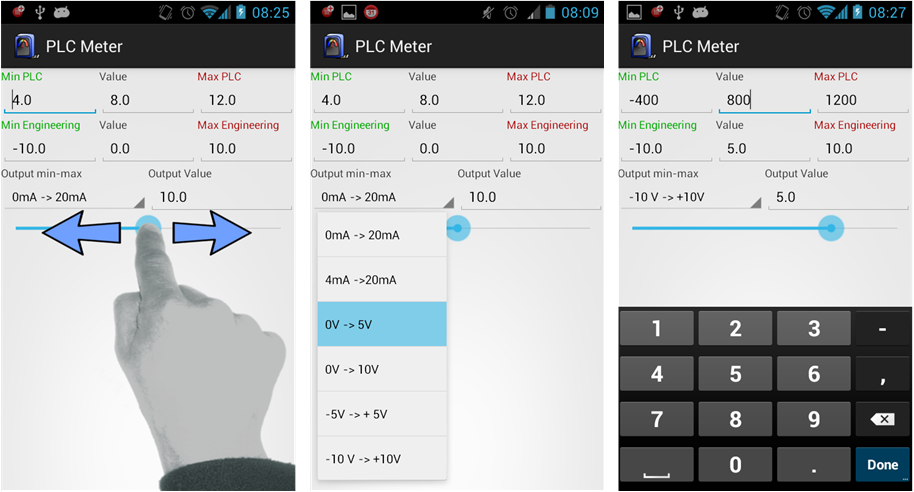# ANALOG SCALING – ANDROID PLC METERDuring my work with programming and diagnostics of PLC controllers, I used several programs installed on my smartphone with Android. Although, the popularity of these tools is not big, it makes our work so much easier.

Sometimes it can happen that we have to count the analog scaling in some PLC program. So, we take the sheet of paper, pen and we count. If you have a phone with Android system, then PLC Meter program will be helpful.

#### PLC METER – analog scaling calculator

PLC METER is very simple tools for testing the analog scaling in PLC controllers. So basically, the program makes it possible to edit y=f(x) function. Also, the method used here, makes that this program is very useful tool for PLC specialists.

The whole program consists of 3 editing fields:

1. Field of analog input of DC converter (Min Max PLC).
2. Range of values / measurement value of the sensor (Min Max Engineering)
3. Analog range of the sensor (Output value)

This program allows for automatic calibration of the value, manually or through usage of slider. If we change one value, then the others will adjust according to the function.

#### Example – analog scaling:

Devices:

• PLC S7-1200 controller + analog input SB1231 AI1 (6ES7 231-4HA30-0XB0),
• Pressure sensor with 4 … 20 mA current output with range of 0 … 16 bar.

Min Max PLC / range of digital converter:

Current representation of analog input in S7-1200 controller:

0mA = 0
4mA = 5530
20mA = 27648

Min Max Engineering

Measurement value of pressure sensor, in this example, is 0 … 16 bar.

Output Value / Sensor analog output:

Analog output of pressure sensor, in this example, is 4 … 20mA.

So in the PLC Meter program we should chose the following values:

At the above example you can see that if the current value on the pressure sensor output is 11,36mA, then it should indicate the value of 7,36 bar.